Vignette 20
Magic Squares

An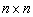normal magic square is an arrangement of the numbers 1, 2, 3, ... n2 in a square array, with the property that the sum of every row and column, as well as both diagonals, is the same number.  An example of anormal magic square is

 6 1 8 7 5 3 2 9 4

You can verify that each of the three rows, the three columns, and the two diagonals add to 15.

Magic Squares in History

Magic squares have been studied for at least three thousand years, the earliest recorded appearance dating to about 2200 BC, in China.  In the 9th century, Arab astrologers used them in calculating horoscopes, and by 1300 AD, magic squares had spread to the West.  An engraving by the German artist Albrecht Dürer included a magic square in which the artist embedded the date, 1514, in the form of two consecutive numbers in the bottom row!  Because the concept of a magic square is so easily understood, magic squares have been particularly attractive to puzzlers and amateur mathematicians.  Even Benjamin Franklin dabbled with magic squares, and an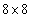magic square with some very interesting properties is attributed to him.

What is the Magic Sum?

Given annormal magic square, suppose M is the number that each row, column and diagonal must add up to.  Then since there are n rows the sum of all the numbers in the magic square must be.  But the numbers being added are 1, 2, 3, ... n2 , and so 1 + 2 + 3 + ... + n2.  In summation notation,.  Using the formula for this sum, we have, and then solving for M gives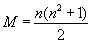.  Thus, anormal magic square must have its rows, columns and diagonals adding to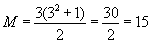, a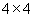to M = 34, Benjamin Franklin'sto M = 260, and so on.

The magic sum for annormal magic square can be found by filling thesquare with the numbers 1, 2, 3, ... n2 -- first going across the top row, then the second row, and so on -- and then adding the numbers along either of the diagonals.   For instance, to find the magic sum of anormal magic square, we form the following square:

 1 2 3 4 5 6 7 8 9 10 11 12 13 14 15 16

and then compute 1 + 6 + 11 + 16 = 34.  See if you can figure out why this works for anynormal magic square.

Creating New Magic Squares from Old Ones

As defined above, a normal magic square uses the numbers 1, 2, 3, ... n2 .  Some people relax this restriction to permit any positive integers, calling the resulting square simply a magic square -- without the adjective "normal."  It is easy obtain magic squares of this generalized type from an existing magic square.  One way is simply to multiply every number used by some positive constant, and/or add a positive constant to every number used.  It should be easy for you to see why this will always work.

A more interesting problem, however, concerns the process of creating normal magic squares.  For odd values of n, there is a simple procedure for constructing a normal magic square.  This procedure is known by several names, including "de la Loubere's algorithm," "the staircase method," and the "Siamese method."  The procedure is illustrated here for the construction of anormal magic square, but it works for any odd value of n.

de la Loubere's Algorithm

Begin by placing a 1 in the middle location of the top row:

 1

We then write successive integers in an upward-right diagonal path, with the following special cases:

• When this upward-right movement would result in a location outside the boundaries of the square, we place the new number at the opposite end of the row or column that would contain the new number, if the rows and columns were not bounded.  (In the case of the current number being in the topmost, rightmost corner, we would try to place the next number in the lowest, leftmost corner.)
• If the upward-right square is already occupied, place the new number directly below the current one.
In thecase, this gives us the following sequence of placements:

 1

 1 2

 1 3 2

 1 3 4 2

 1 3 5 4 2

 1 6 3 5 4 2

 1 6 3 5 7 4 2

 8 1 6 3 5 7 4 2

 8 1 6 3 5 7 4 9 2

Now see if you can follow this algorithm to build a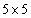normal magic square.

There is no known algorithm for generating even-order normal magic squares -- that is,normal magic squares where n is even.  However, there are some methods for creating magic squares of specific even orders.  For example, we can generate a normalmagic square in the following way.  Begin with thesquare in which the numbers 1 through 16 are listed across the rows:

 1 2 3 4 5 6 7 8 9 10 11 12 13 14 15 16

Then interchange numbers on the diagonals that are symmetrically located with respect to the center.  (In the square above, we will interchange the numbers that are identically colored.)  The result is anormal magic square:

 16 2 3 13 5 11 10 8 9 7 6 12 4 14 15 1

This process works only for thenormal magic square, however.

How Many Magic Squares Are There?

Of course, given any magic square, rotating it or reflecting it will produce another magic square.  Not counting these as distinct, it is known that there is only onenormal magic square, and there are 880normal magic squares.  The number of normal magic squares increases dramatically as the size of the square increases.  For instance, there are over 13 million normalmagic squares!

Further Exploration